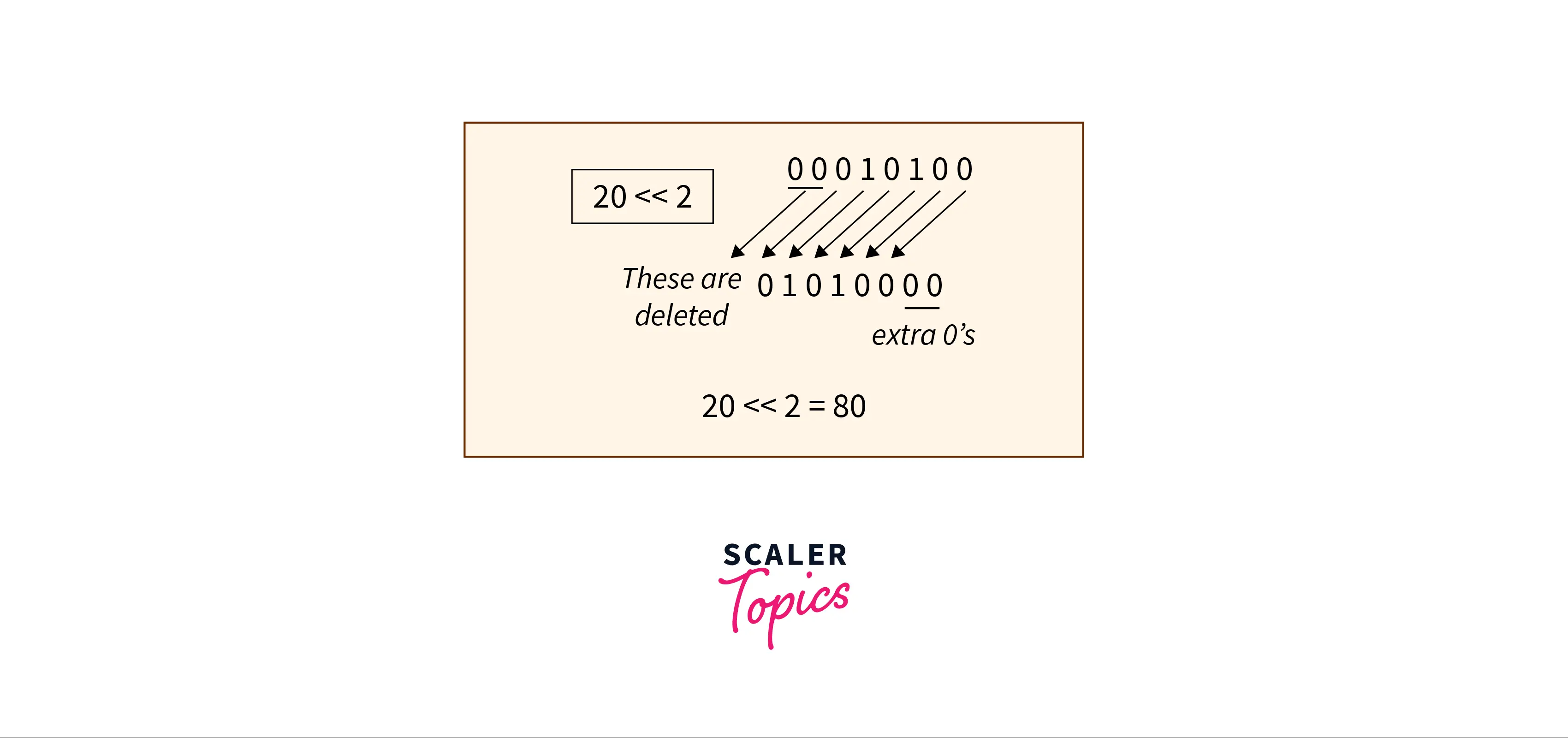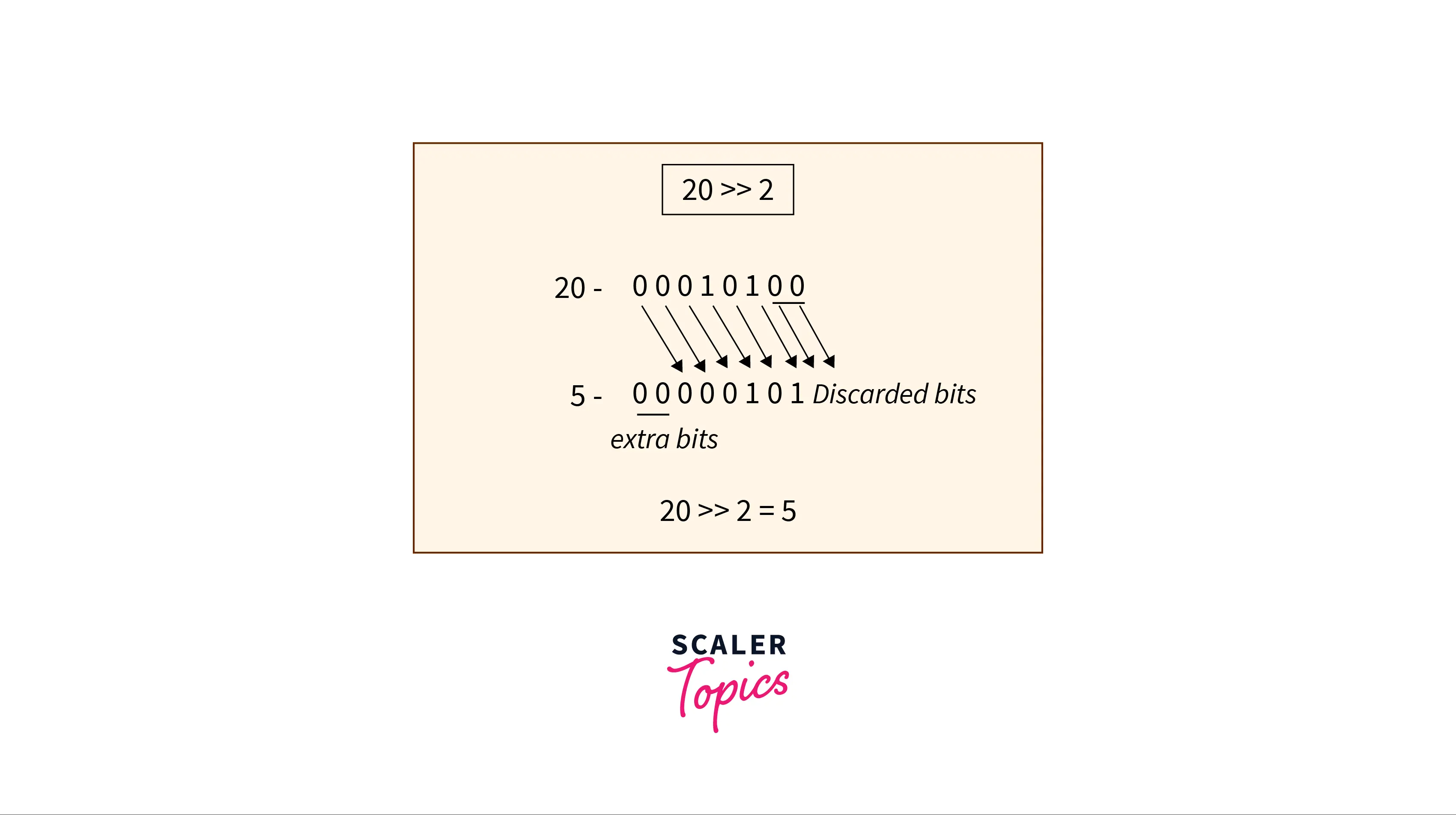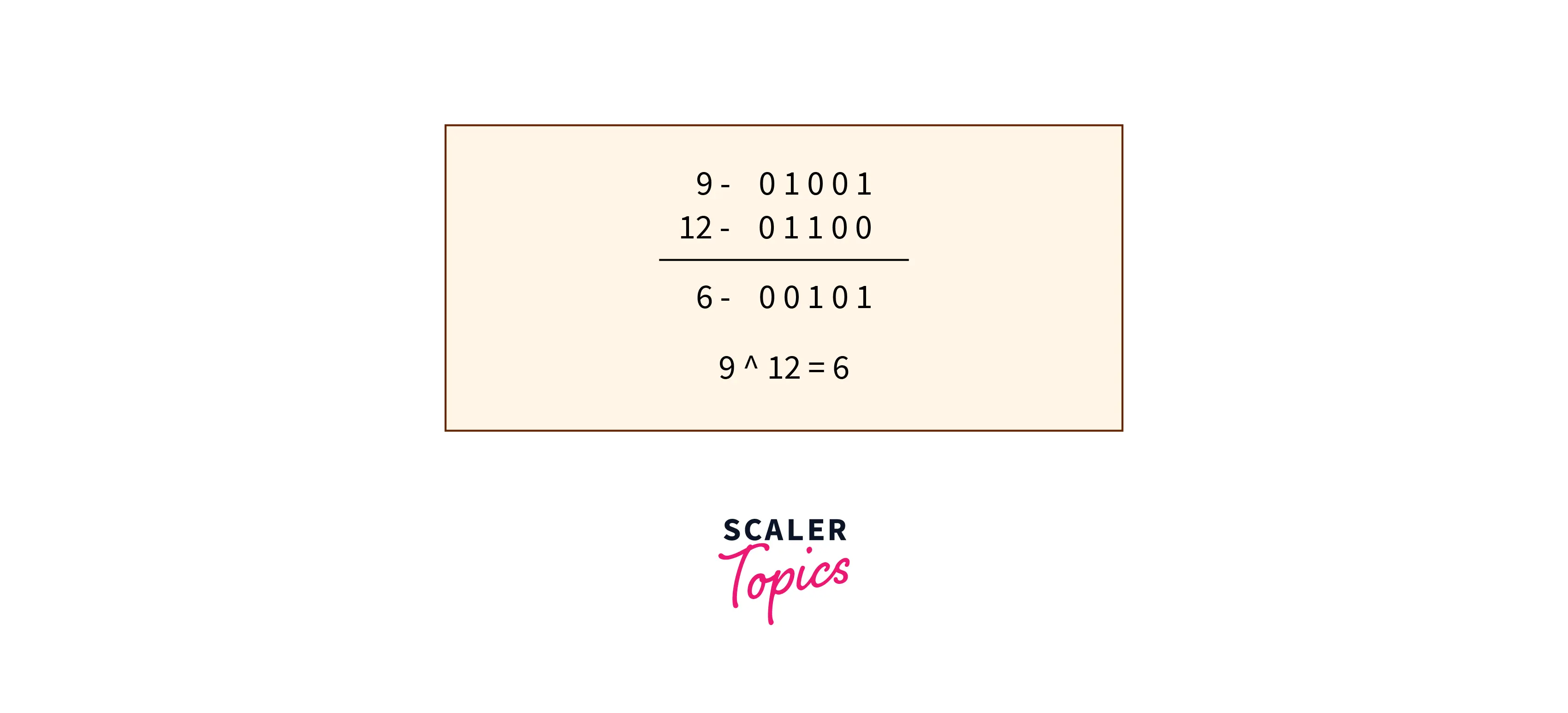# JavaScript Math random() Method

Challenge Inside! : Find out where you stand! Try quiz, solve problems & win rewards!
Learn via video courseJavaScript Course With Certification: Unlocking the Power of JavaScript
By Mrinal BhattacharyaFree
4.8Enrolled: 1000JavaScript Course With Certification: Unlocking the Power of JavaScript
Mrinal BhattacharyaFree
4.8
Enrolled: 1000

## Overview

While solving programming problems we want to add some randomness to the solutions we design. This can be the case if you are building an application for Casino or some other online lucky draw app, then you surely want things to get 'random'.

To enable this functionality, random numbers come into the picture. As we know that computers are good at maths and thus they can easily generate random numbers.

In this article, you will learn how you can generate random numbers with JavaScript using the Math.random() method.

## Syntax of JavaScript Math random() Method

Well, as with most other things in JavaScript using the Math.random() method is quite simple

Here, Math is the name of the class and random is the method name.

## Parameters of JavaScript Math random() Method

The Math random javascript method in JavaScript does not need any arguments.

## Return Value of JavaScript Math random() Method

When you call this method it will give a pseudo-random number between 0 and 1.

NOTE : In the output 0 is inclusive and 1 is exclusive. This means the output can have 0 but it will not have 1.

## Exceptions of JavaScript Math random() Method

Math random JavaScript method does not have any exceptions.

## Example

Output:

The output of this code is not fixed, of course, you generating a random number you do not want it to be the same.

## How does the JavaScript Math random() Method work?

An interesting fact about random numbers: The computers we use are finite state machines which means that their outcomes are deterministic and theoretically we should not be able to generate random numbers.

But I have seen an app that gives me random numbers every time! How is this possible then? You are not wrong, but also not 100% right. Let me explain.

Numbers that our computers generate are called pseudo-random numbers. While these numbers CAN repeat, that doesn't mean they do so consistently. Pseudo-random numbers are good enough for our practical applications. The algorithms that generate these numbers make them very hard to repeat so we do not need to worry about the random number is "not random".

The algorithms do a good job of simulating randomness. This is the reason why they are technically called Pseudo-random number generators (PRNGs).

When we use math to create a sequence of numbers, these numbers may initially appear random, but gradually they will repeat and reveal a pattern that is not random.

The PRNG is an algorithm and one PRNG can be better than the other. These PRNG use different factors to generate numbers one of the factors being period which is how many iterations a PRNG undergoes before it begins to repeat itself.

You might be wondering what PRNG JavaScript uses. The use of PRNG is not dependent on JavaScript; rather, it is dependent on the browser. Which PRNG JavaScript will use is determined by the engineers who design our browser.

One famous algorithm is xorshift128+. To give some idea of how this algorithm works, it uses 3 bitwise operations:

• Left Shift
• Right Shift
• XOR

### Left Shift (<<)

Left shift is a bitwise operation that shifts all the bits to the left.

Example:### Right Shift (>>)

Right shift is a bitwise operation that shifts all the bits to the right.

Example:### XOR (^)

XOR stands for Exclusive OR and this operation compares the binary representation of two numbers. The output is 1 if both the bits are different and 0 if both are the same.

Example:xorshift128+ uses these operations to evaluate the expression similar to this s1 ^= s1 << 23. This is a very oversimplified version but this gives an idea of how the algorithm works.

## More Examples

### Generate Random Numbers Between Two Numbers

To generate random numbers in a range of numbers we can multiply the output of the Math.random() method by the difference between those numbers and then we add the smaller number.

Output:

### Generate a Random Integer Between Two Numbers

If we want to generate a random integer between two given numbers then we can use the code used above and can use Math.floor() on the result we get from Math.random().

Output:

### Generate Integer between Two Numbers(inclusive)

To generate random numbers between two numbers and including both the min and the max number we can code

Output:

## Supported Browsers

Math random JavaScript method is supported by all Desktop, Mobile browsers, and Runtime Environments (Node and Deno).

SupportedVersion (Full Support)
Chrome1
Firefox1
Edge12
Internet Explorer3
Safari1
Chrome Android18